# Operational Transconductance Amplifier

## What is Current Conveyors (CCs)?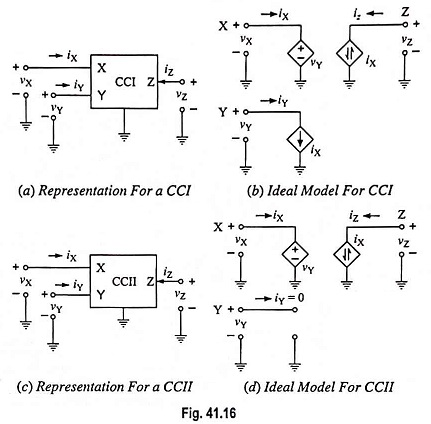What is Current Conveyors (CCs)? Current conveyors (CCs) were first introduced by Smith and Sedra and have been used in a variety of electronic applications. The CC is a three-port device described by the hybrid equations in which symbols for the port variable represent total instantaneous quantities. a = 1 represents the first generation conveyor, …

## OTA Based Ground Inductor and Floating Inductor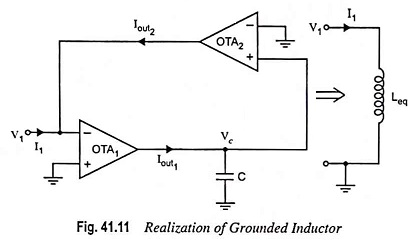OTA Based Ground Inductor and Floating Inductor: The OTA Based ground inductor can be realized by using 2 OTAs as shown in Fig. 41.11. Using Eq. (41.1), we have and Also we have where Vc is the voltage across capacitor and is given as Substituting Vc = I1/gm2 from Eq. (41.28), we have From Eqs. (41.26) and …

## OTA as Programmable Resistor, Grounded Resistor and Floating Resistor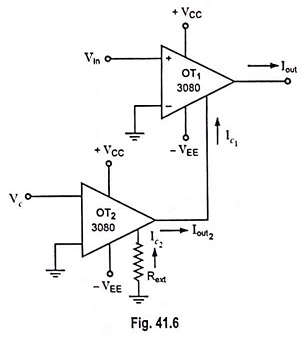OTA as Programmable Resistor, Grounded Resistor and Floating Resistor: OTA as a Programmable Resistor: We may employ Ic OTA as a programmable resistor whose resistance is controlled by controlling current Ic. We can define resistance, using Eq. (41.16) Two OTAs can be employed to behave as programmable resistor, as shown in Fig. 41.6. Now to …

## OTA Voltage Amplifier Circuit Diagram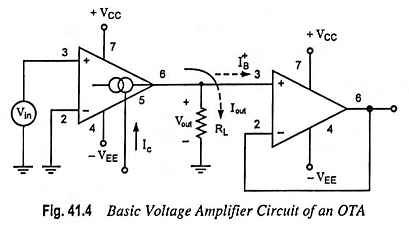OTA Voltage Amplifier Circuit Diagram: A basic OTA voltage amplifier circuit diagram is shown in Fig. 41.4. At room temperature (T = 300 K), the output current, Now, if the whole output current Iout flows into the RL. This will occur only if [IB+ ≪ Iout], thus Thus, the RL also contributes to voltage gain. And …

## Operational Transconductance Amplifier (OTA)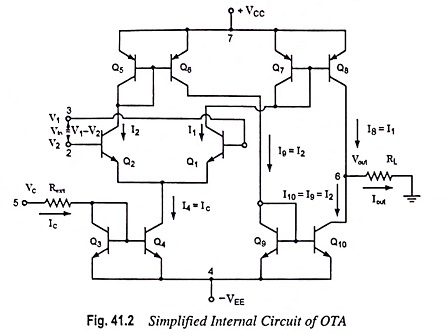Operational Transconductance Amplifier (OTA): The operational amplifiers considered up to this point have been voltage amplifiers. The input signal is a voltage and the output signal is a voltage. Another type of op-amp is an operational transconductance amplifier (OTA) which produces an output current proportional to an input voltage. The constant of proportionality is the …

Scroll to Top